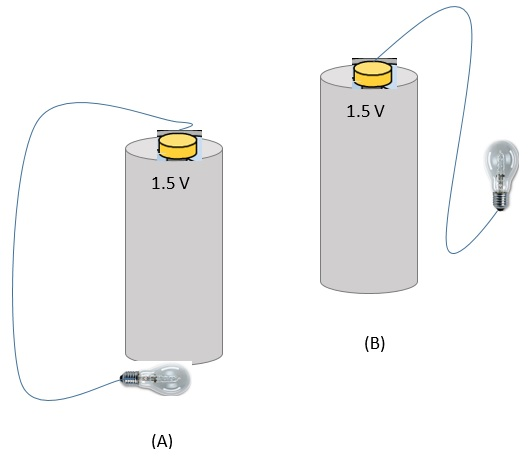# Introduction to Conductors, Insulators, and Electric Circuits

## Conductors and Insulators

### Conductors

• A conductor is any material that has loose electrons in the outer atomic shells.
• Loose, basically, means the electrons become detached from the nuclei and are free to move around within the material.
• The material as a whole remains electrically neutral.
• Examples: our bodies, metals such as copper and aluminum.

### Insulators

• An insulator is any material where the electrons are not loose.
They are tightly bound to the nuclei and are not free to move around.
• Examples: rubber and glass.
• Insulators are used to protect us from conductors.
For example, wires and power cords (conductors) used at home are covered with plastic (insulator).

## Electric Circuits

Charges will tend to flow from one terminal to the other if an external conducting path, i.e. circuit, is provided.
In addition to an energy source and a conducting path, a circuit also includes some resistance to the current.
Electric current (I) is then the flow of electric charge.
• In metal wires, electrons make up the flow of charge.
• In conducting fluids, such as a car battery, positive ions and electrons make up the flow of electric charge.
• I = q / t where I is electric current, q is charge, and t is time.
• The standard unit for electric current is the ampere: 1 A = 1 C / s
By definition, the direction of the electric current is the direction of flow of positive charge.
The flow is measured with respect to time. Hence, electric current is the rate of flow of electric charge.
Where,
• I is the symbol for electric current measured in amperes,
• q is the charge measured in coulomb, and
• t is time measured in seconds.
Example 1:
Assume 6 C of charge flow through a wire in 4 s, then the electric current I is 6 C / 4 s = 1.5 A.

Example 2:
Two arrangements of a battery, bulb, and wire are shown below.Question: Which of the two arrangements will light the bulb?